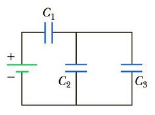Chapter 16, Problem 44P

Chapter
Section
Textbook Problem

Three capacitors are connected to a battery as shown in Figure P16.44. Their capacitances are C1 = 3C, C2 = C, and C3 = 5C. (a) What is the equivalent capacitance of this set of capacitors? (b) State the ranking of the capacitors according to the charge they store from largest to smallest. (c) Rank the capacitors according to the potential differences across them from largest to smallest. (d) Assume C3 is increased. Explain what happens to the charge stored by each capacitor.Figure P16.44

(a)

To determine
The equivalent capacitance.

Explanation

The capacitors C2 and C3 are connected in parallel combination. The equivalent capacitance is,

Cp=C2+C3

The capacitances Cp and C1 are in series combination. The total equivalent capacitance is,

Ceq=CpC1Cp+C1

Therefore,

Ceq=C1(C2+C3)C1+C2+

(b)

To determine
The charge on each capacitor.

(c)

To determine
The potential difference on each capacitor.

(d)

To determine
The effect of increasing C3

Still sussing out bartleby?

Check out a sample textbook solution.

See a sample solution

The Solution to Your Study Problems

Bartleby provides explanations to thousands of textbook problems written by our experts, many with advanced degrees!

Get Started

Find more solutions based on key concepts